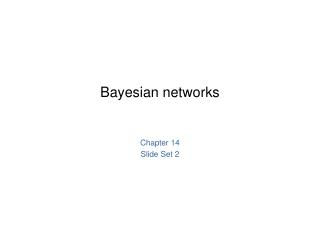DownloadDownload PresentationBayesian networks

# Bayesian networks

Télécharger la présentation## Bayesian networks

- - - - - - - - - - - - - - - - - - - - - - - - - - - E N D - - - - - - - - - - - - - - - - - - - - - - - - - - -
##### Presentation Transcript

1. Bayesian networks Chapter 14 Slide Set 2

2. Constructing Bayesian networks • 1. Choose an ordering of variables X1, … ,Xn • 2. For i = 1 to n • add Xi to the network • select parents from X1, … ,Xi-1 such that P (Xi | Parents(Xi)) = P (Xi | X1, ... Xi-1)

3. Example • Suppose we choose the ordering M, J, A, B, E P(J | M) = P(J)?

4. Example • Suppose we choose the ordering M, J, A, B, E P(J | M) = P(J)? No P(A | J, M) = P(A | J)?P(A | J, M) = P(A)?

5. Example • Suppose we choose the ordering M, J, A, B, E P(J | M) = P(J)? No P(A | J, M) = P(A | J)?P(A | J, M) = P(A)? No P(B | A, J, M) = P(B | A)? P(B | A, J, M) = P(B)?

6. Example • Suppose we choose the ordering M, J, A, B, E P(J | M) = P(J)? No P(A | J, M) = P(A | J)?P(A | J, M) = P(A)? No P(B | A, J, M) = P(B | A)? Yes P(B | A, J, M) = P(B)? No P(E | B, A ,J, M) = P(E | A)? P(E | B, A, J, M) = P(E | A, B)?

7. Example • Suppose we choose the ordering M, J, A, B, E P(J | M) = P(J)? No P(A | J, M) = P(A | J)?P(A | J, M) = P(A)? No P(B | A, J, M) = P(B | A)? Yes P(B | A, J, M) = P(B)? No P(E | B, A ,J, M) = P(E | A)? No P(E | B, A, J, M) = P(E | A, B)? Yes

8. Example contd. • Deciding conditional independence is hard in noncausal directions • (Causal models and conditional independence seem hardwired for humans!) • Network is less compact: 1 + 2 + 4 + 2 + 4 = 13 numbers needed

9. Using a Bayesian Network Suppose you want to calculate: P(A = true, B = true, C = true, D = true) = P(A = true) * P(B = true | A = true) * P(C = true | B = true) P( D = true | B = true) = (0.4)*(0.3)*(0.1)*(0.95) A B C D

10. Using a Bayesian Network Example Using the network in the example, suppose you want to calculate: P(A = true, B = true, C = true, D = true) = P(A = true) * P(B = true | A = true) * P(C = true | B = true) P( D = true | B = true) = (0.4)*(0.3)*(0.1)*(0.95) This is from the graph structure A B These numbers are from the conditional probability tables C D

11. Inference • Using a Bayesian network to compute probabilities is called inference • In general, inference involves queries of the form: P( X | E ) E = The evidence variable(s) X = The query variable(s)

12. Inference HasAnthrax • An example of a query would be: P( HasAnthrax = true | HasFever = true, HasCough= true) • Note: Even though HasDifficultyBreathing and HasWideMediastinum are in the Bayesian network, they are not given values in the query (ie. they do not appear either as query variables or evidence variables) • They are treated as unobserved (hidden) variables HasCough HasFever HasDifficultyBreathing HasWideMediastinum

13. The Bad News • Exact inference in BBNs is NP-hard • Though feasible for singly-connected networks • But we can achieve significant improvements (e.g., variable elimination) • There are approximate inference techniques which are much faster and give fairly good results • Next class: inference

14. Example • In your local nuclear power plant, there is an alarm that senses when a temperature gauge exceeds a given threshold. The gauge measures the temperature of the core. Consider the Boolean variables: A (alarm sounds), FA (alarm faulty), FG (gauge is faulty), and the multivalued variables G (gauge reading) and T (actual core temperature. • The gauge is more likely to fail when the core temperature gets too high • Let’s draw the network (in class)

15. Example (cont) • Suppose • there are just two possible actual and measured temperatures, normal and high • The prob that the gauge gives the correct temp is X when it is working, but Y when it is faulty. Give the CPT for G (in class) • Suppose • The alarm works correctly unless it is faulty, in which case it never sounds. Give the CPT for A (in class)

16. Example (cont.) • Suppose FA=false; FG=false (the alarm and gauge are working properly); and A=True (and the alarm sounds). What is the probability that the temperature is too high? (T=high?) (in class)